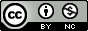Objectives

• Monthly time series often exhibit seasonal variation. January data are similar to observations at a different January, etc.

• Many time series exhibit a trend.

• We wish to extend the theoretical and practical elegance of the ARMA framework to cover these situations.

## 6.1 Seasonal autoregressive moving average (SARMA) models

• A general SARMA$$(p,q)\times(P,Q)_{12}$$ model for monthly data is

[S1] $${\quad\quad\quad}{\phi}(B){\Phi}(B^{12}) (Y_n-\mu) = {\psi}(B){\Psi}(B^{12}) \epsilon_n$$,

where $$\{\epsilon_n\}$$ is a white noise process and $\begin{eqnarray} \mu &=& {\mathbb{E}}[Y_n] \\ {\phi}(x)&=&1-{\phi}_1 x-\dots -{\phi}_px^p, \\ {\psi}(x)&=&1+{\psi}_1 x+\dots +{\psi}_qx^q, \\ {\Phi}(x)&=&1-{\Phi}_1 x-\dots -{\Phi}_px^P, \\ {\Psi}(x)&=&1+{\Psi}_1 x+\dots +{\Psi}_qx^Q. \end{eqnarray}$

• We see that a SARMA model is a special case of an ARMA model, where the AR and MA polynomials are factored into a monthly polynomial in $$B$$ and an annual polynomial in $$B^{12}$$. The annual polynomial is also called the seasonal polynomial.

• Thus, everything we learned about ARMA models (including assessing causality, invertibility and reducibility) also applies to SARMA.

• One could write a SARMA model for some period other than 12. For example, a SARMA$$(p,q)\times(P,Q)_{4}$$ model could be appropriate for quarterly data. In principle, a SARMA$$(p,q)\times(P,Q)_{52}$$ model could be appropriate for weekly data, though in practice ARMA and SARMA may not work so well for higher frequency data.

• Consider the following two models:

[S2] $${\quad\quad\quad}Y_n = 0.5 Y_{n-1} + 0.25 Y_{n-12} + \epsilon_n$$,

[S3] $${\quad\quad\quad}Y_n = 0.5 Y_{n-1} + 0.25 Y_{n-12} - 0.125 Y_{n-13} + \epsilon_n$$,

### 6.1.2 Question: Why do we assume a multiplicative structure in [S1]?

• What theoretical and practical advantages (or disadvantages) arise from requiring that an ARMA model for seasonal behavior has polynomials that can be factored as a product of a monthly polynomial and an annual polynomial?

### 6.1.3 Fitting a SARMA model

• Let’s do this for the full, monthly, version of the Lake Huron data described in Section 5.5.

• First, we’ll revisit reading in the data.

system("head huron_depth.csv",intern=TRUE)
##   "# downloaded on 1/24/16 from\r"
##   "# http://www.glerl.noaa.gov/data/dashboard/data/levels/mGauge/miHuronMog.csv\r"
##   "# Lake Michigan-Huron:, Monthly Average Master Gauge Water Levels (1860-Present)\r"
##   "# Source:, NOAA/NOS\r"
##   "Date, Average\r"
##   "01/01/1860,177.285\r"
##   "02/01/1860,177.339\r"
##   "03/01/1860,177.349\r"
##   "04/01/1860,177.388\r"
##  "05/01/1860,177.425\r"
dat <- read.table(file="huron_depth.csv",sep=",",header=TRUE)
dat$Date <- strptime(dat$Date,"%m/%d/%Y")
dat$year <- as.numeric(format(dat$Date, format="%Y"))
dat$month <- as.numeric(format(dat$Date, format="%m"))
head(dat)
##         Date Average year month
## 1 1860-01-01 177.285 1860     1
## 2 1860-02-01 177.339 1860     2
## 3 1860-03-01 177.349 1860     3
## 4 1860-04-01 177.388 1860     4
## 5 1860-05-01 177.425 1860     5
## 6 1860-06-01 177.461 1860     6
huron_depth <- dat$Average time <- dat$year + dat\$month/12 # Note: we treat December 2011 as time 2012.0, etc
plot(huron_depth~time,type="l")